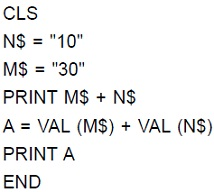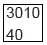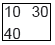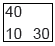# User Forum

Subject :NCO    Class : Class 7

What would be the output of the given QBASIC program?ABCDWHAT DOES "VAL" COMMAND MEANS ?????

## Ans 1:

Class : Class 7
According to the question, the value you put in the "X" are not used for mathematical operations, therefore answer will be 3010 but when you right val it means value of the object in "X" so "30" + "10" will be 3010 but Val (30) + Val (10)= 40 answer is a

## Ans 2:

Class : Class 8
In this programme, the VAL means the original value of the numericals.As, M\$=30 N\$=10 A=VAL(M\$)+VAL(N\$) So, VAL(M\$)=30 VAL(N\$)=10 The '+' sign is used as 'space'. In this programme, it does not indicate addition function because of the "VAL". So, the answer is 30 10